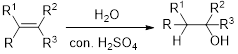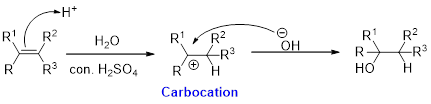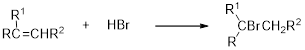Chapter 23, Problem 79GQ

Chapter
Section
Textbook Problem

Write equations for the reactions of cis-2-butene with the following reagents, representing the reactants and products using structural formulas.(a) H2O(b) HBr(c) Cl2

(a)

Interpretation Introduction

Interpretation:

The reactions is to be written for the reaction of cis-2-butene with H2O reagents.

Concept Introduction:

Hydration:

When alkene is undergoes hydration with water in the presence of sulfuric acid which yields the alcohol. In this reaction, the water molecule will behave like a hydrogen halide to the alkene which gives the addition product this reaction is known as a hydration reaction.Alkene is reaction with water in the presence of sulfuric acid, first step is proton (H+) addition to the double bond which forms carbocation (more stable carbocation) followed by addition of hydroxide ion (-OH) yields alcohol as a product. The stability of carbocation is given below, Tertiary carbocation is more stable than the secondary, secondary carbocation is more stable than primary.In hydration reaction, sulfuric acid is act as a proton donor, which is the driving force of the reaction. Hydration reaction will not go without acid (sulfuric acid).

Explanation

The reaction of cis-2-butene with H2O is given below.

(b)

Interpretation Introduction

Interpretation:

The reactions is to be written for the reaction of cis-2-butene with HBr reagents.

Concept Introduction:

Hydrobromination:

A hydrobromination reaction is one of the electrophilic additions to alkenes to yield the corresponding bromo alkanes. In this reaction the bromine atom adds to the double bond carbon which is having lesser number of hydrogen or more substituted carbon (Markovnikov's rule).(b)

Interpretation Introduction

Interpretation:

The reaction is to be written for the reaction of cis-2-butene with Cl2 reagents.

Concept Introduction:

An alkene undergo halogenation reaction when it is treated with halogens. The addition of two halogen atoms takes place on the carbon-carbon double bond. The product formed is called as disubstituted haloalkanes.

Still sussing out bartleby?

Check out a sample textbook solution.

See a sample solution

The Solution to Your Study Problems

Bartleby provides explanations to thousands of textbook problems written by our experts, many with advanced degrees!

Get Started